# 6.3 Summarizing the Models

For discretization the one-dimensional projections of eqns. (5.38) and (5.40) onto a direction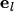has to be considered: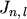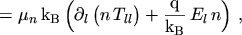(6.16)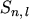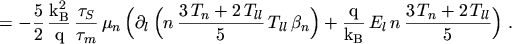(6.17)

By assuming an isotropic MAXWELLIAN distribution, which results in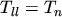and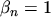, the conventional energy transport model is obtained.

The carrier temperature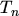defined by eqn. (2.94) is a measure of average carrier energy. The diagonal component of the temperature tensor is given by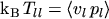. Off-diagonal components are neglected. The solution variable is still the carrier temperature, whereas the tensor components and the fourth order moment are modeled empirically as functions of(enqs. (6.3) and (6.15)):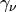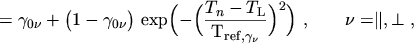(6.18)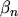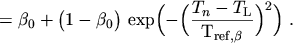(6.19)

The empirical model for the temperature tensor distinguishes between directions parallel () and normal (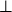) to the current density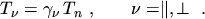(6.20)

The diagonal temperature for a generic directionis obtained from the average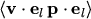after neglecting the off-diagonal terms as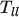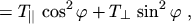(6.21)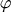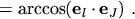(6.22)

The graphs of the functions of eqns. (6.18) and (6.19) are displayed in Fig. 6.7.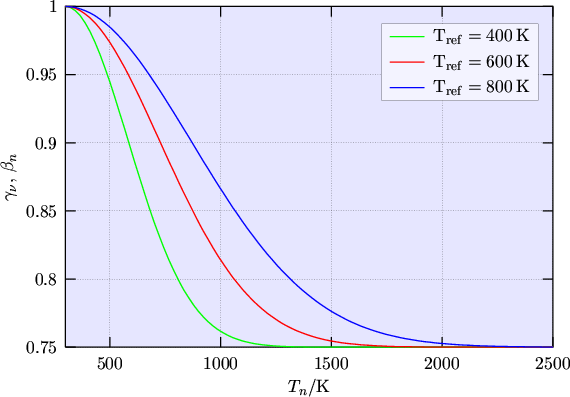Both functions assume unity for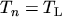and an asymptotic value for large. The exact shape of the transition between these two regions is only of minor importance and mainly affects the numerical stability. Therefore the transition should not be too steep.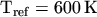appeared to be an appropriate value. Parameter values for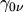,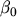, and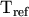can be roughly estimated from Monte Carlo simulations of one-dimensional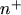-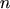-test structures (Tbl. 6.1).

Table 6.1: Parameter values estimated from Monte Carlo simulations.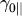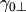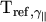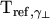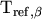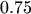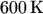Monte Carlo results for the anisotropic temperature in a MOSFET are shown in Fig. 6.8 and Fig. 6.9 in comparison with the analytical models. Fig. 6.8 indicates that values for the anisotropy parameter can be as low as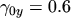. Values close to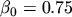for the non-MAXWELLian parameter in the channel region can be estimated from Fig. 6.9. These parameters show only a weak dependence on doping and applied voltage.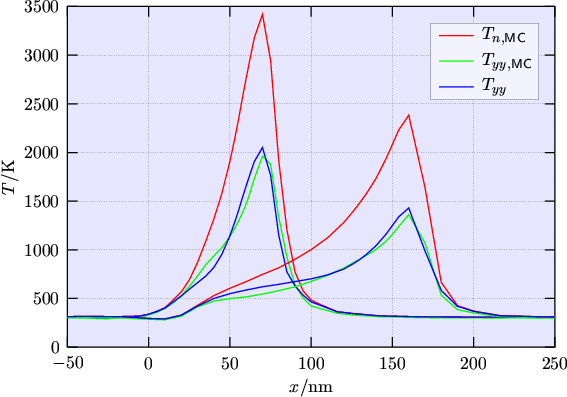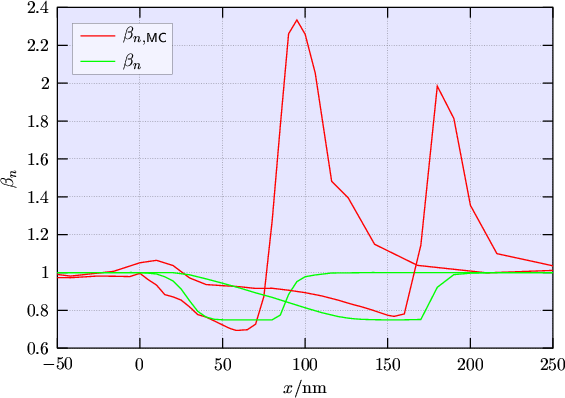M. Gritsch: Numerical Modeling of Silicon-on-Insulator MOSFETs PDF